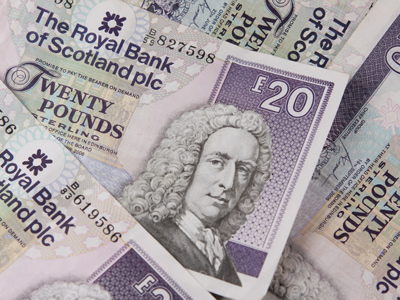What is 1/8 of £20.00?

# Numbers - Fractions - Addition and Subtraction

This Math quiz is called 'Numbers - Fractions - Addition and Subtraction' and it has been written by teachers to help you if you are studying the subject at middle school. Playing educational quizzes is a fabulous way to learn if you are in the 6th, 7th or 8th grade - aged 11 to 14.

It costs only \$12.50 per month to play this quiz and over 3,500 others that help you with your school work. You can subscribe on the page at Join Us

You can only add and subtract fractions if they have the same denominator. Fractions have numerators (the top or first number) and denominators (the bottom or second number).

Practice with this Math quiz all about addition and subtraction of fractions.

1.
What is 1/4 + 1/2?
1/8
2/6
3/4
4/9
2.
What is 3/5 - 1/4?
4/10
4/20
7/10
7/20
3.
How many sixths are there in 323?
12
22
32
42
4.
What is the lowest common multiple of the denominators in 1/4 + 1/2?
1
2
3
4
4 is the lowest common multiple of 4 and 2, therefore change 1/2 into an equivalent fraction with a denominator of 4
5.
What is 1/8 of £20.00?
80p
£1.20
£1.25
£2.50
6.
For the calculation 1/4 + 1/2, what would you change 1/2 into in order to find the answer?
3/4
2/4
1/4
4/6
The lowest common multiple is 4, and 2/4 is an equivalent fraction of 1/2
7.
What is 3/4 - 1/5?
1/20
5/20
11/20
15/20
8.
How many sixths are there in 356?
15
17
23
27
9.
What is the lowest common multiple of the denominators in 3/4 - 1/5?
9
12
18
20
It is the smallest number in the 4 and 5 times table
10.
£6.00 = 1/8 of .......
£48.00
£52.00
£56.00
£62.00
Author:  Frank Evans